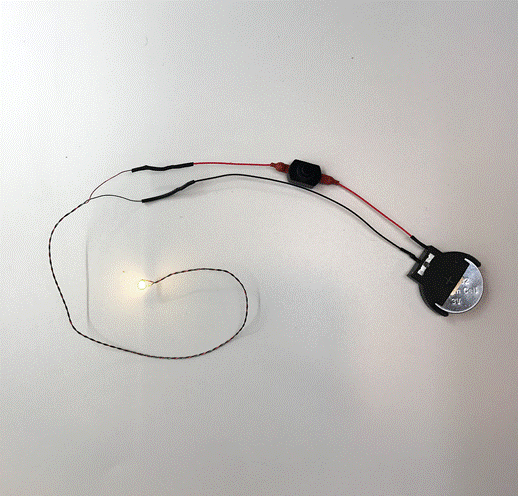# What is Voltage

by Brynna Jamison

## What is Voltage? Learning how Voltage works in a Mini LED Light Kit.

Welcome to Evan Design’s Blog Series on Electricity. We thought we would dive a little deeper into understanding more about the electricity that powers our small LED lights. As you might notice all of our miniature lighting at Evan Designs comes in a variety of different voltage options ranging from 3 volts to 19 volts. Anyone without a background in physics or electronics might be asking themselves “what is voltage anyways”? In this article, we will answer that question.

First, before we can understand what voltage is, we must understand how an electrical circuit works. We will be using an Evan Designs mini LED connected to a 3-volt battery as our circuit example (see Figure A). In this example, our 3-volt battery provides the “current” for our circuit. Current is the rate at which electrons (particles with a negative electrical charge) flow through a circuit. The electrons will flow from our battery to our miniature LED light and this light will use the current to produce light. In short a circuit is a closed path that allows for electricity to flow from one point to another. If you disconnect a small LED wire from the battery, the circuit will be broken, the current will cease to flow and the hobby LED lights will turn off.Figure A: Evan Designs Mini LED light kit, an example of a circuit.

So let's review our vocabulary:

• Circuit: a closed path that allows for electricity to flow from one point to another.
• Current: the rate at which electrons flow through a circuit.
• Electrons: Subatomic particles with a negative (-) electrical charge.

Now that we better understand the circuit created in a LED light kit, we can work to understand voltage. Voltage can be thought of as pressure. Pressure is the amount of force exerted on an object. The 3-volt battery in our LED light kit has a positive and a negatively charged side. We can see this by looking at our battery, one size will have a plus sign on it. In the battery, through electrochemical reactions, the electrons become negatively charged. They then flow out through the circuit to the positively charged end of the hobby lights. By illuminating the battery-powered LED lights the electrons lose their negative charge and flow back to the positive end of the battery. This is illustrated in Figure B below.Figure B: Lighting Circuit Diagram

The potential energy difference in the battery exerts a force on charged electrons inside the battery and then pushes them through the circuit, creating pressure. The higher the voltage of our battery the more pressure it will exert on the electrons and the more current will flow through our circuit. We use the unit volts to measure the voltage (pressure) of our battery.

In short:

• Voltage: the pressure from the 3-volt battery pushing electrons through our circuit.
• Pressure: the amount of force exerted on an object.
• Volts: the unit of measure for voltage.

Using this information, let’s learn more about what the different voltages of our small LED lights mean. Our Evan Designs LEDs range from 3 volts to 19 volts. You may be asking yourself what’s the difference between 3 volts and 9 volts vs 19 volts. The higher voltage of the battery simply means that the battery has more electrons in it and a higher potential energy difference between the + and - of the battery. Due to this the battery can exert more pressure on the electrons, and push them faster through the circuit. Therefore, it is important to match the voltage of your battery to your miniature LED light. If too many electrons are pushed too rapidly to your light, ie. using a 3 volt light on a 9-volt battery your hobby lights will burn out.